# Basic Concepts of Three Dimensional JEE Notes | EduRev

## JEE : Basic Concepts of Three Dimensional JEE Notes | EduRev

The document Basic Concepts of Three Dimensional JEE Notes | EduRev is a part of the JEE Course Mathematics (Maths) Class 11.
All you need of JEE at this link: JEE

Distance Between Two Points
Let P and Q be two given points in space. Let the co-ordinates of the points P and Q be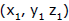and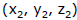with respect to a set OX, OY, OZ of rectangular axes.

The position vectors of the points P and Q are given by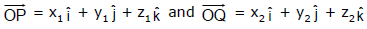Now we have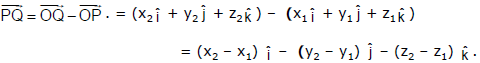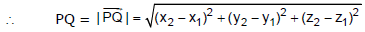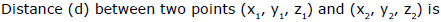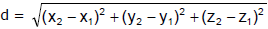B. Section Formula

1.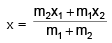2.3.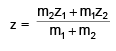(for external division take –ve sign)
To determine the co-ordinates of a point R which divides the joining of two points P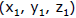and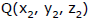internally in the ratio m1 : m2. Let OX, OY, OZ be a set of rectangular axes.
The position vectors of the two given points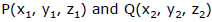are given by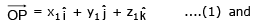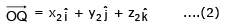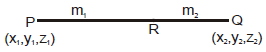Also if the co-ordinates of the point R are (x, y, z), then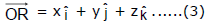Now the point R divides the join of P and Q in the ratio m1 : m2, so that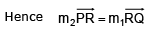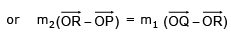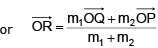or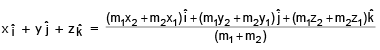[Using (1), (2) and (3)]

Remark : The middle point of the segment PQ is obtained by putting m1 = m2. Hence the co-ordinates of the middle point of PQ are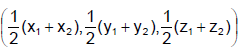Centroid Of A Triangle:

Let ABC be a triangle. Let the co-ordinates of the vertices A, B and C beand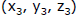respectively. Let AD be a median of the ΔABC. Thus D is the mid point of BC.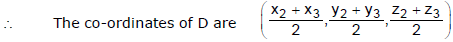Now if G is the centroid of ΔABC, then G divides AD in the ratio 2 : 1. Let the co-ordinates of G be (x, y, z).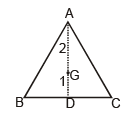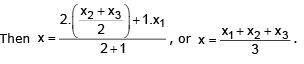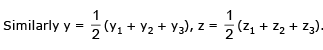Centroid Of A Tetrahedron :

Let ABCD be a tetrahedron, the co-ordinates of whose vertices are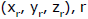= 1, 2, 3, 4.

Let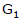be the centroid of the face ABC of the tetrahedron. Then the co-ordinates ofare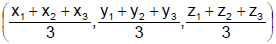The fourth vertex D of the tetrahedron does not lie in the plane of ΔABC. We know from statics that the centroid of the tetrahedron divides the line DG1 in the ratio 3 : 1. Let G be the centroid of the tetrahedron and if (x, y, z) are its co-ordinates, then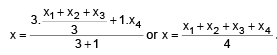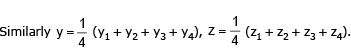Ex.1 P is a variable point and the co-ordinates of two points A and B are (–2, 2, 3) and (13, –3, 13) respectively. Find the locus of P if 3PA = 2PB.

Sol. Let the co-ordinates of P be (x, y, z).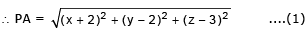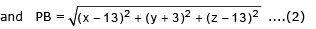Now it is given that 3PA = 2PB i.e., 9PA2 = 4PB2. ....(3)

Putting the values of PA and PB from (1) and (2) in (3), we get

9{(x + 2)2 + (y – 2)2 + (z – 3)2} = 4 {(x – 13)2 + (y + 3)2 + (z – 13)2}

or 9 {x2 + y2 + z2 + 4x – 4y – 6z + 17} = 4{x2 + y2 + z2 – 26x + 6y – 26z + 347}

or 5x2 + 5y2 + 5z2 + 140x – 60 y + 50 z – 1235 = 0

or x2 + y2 + z2 + 28x – 12y + 10z – 247 = 0

This is the required locus of P.

Ex.2 Find the ratio in which the xy-plane divides the join of (–3, 4, –8) and (5, –6, 4). Also find the point of intersection of the line with the plane.

Sol. Let the xy-plane (i.e., z = 0 plane) divide the line joining the points (–3,4, –8) and (5, –6, 4) in the ratio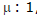,  in the point R. Therefore, the co-ordinates of the point R are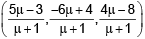....(1)

But on xy-plane, the z co-ordinate of R is zero

(4μ - 8) / (μ + 1) = 0, or μ = 2. Hence  μ : 1 = 2 : 1. Thus the required ratio is 2 : 1.
Again putting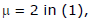the co-ordinates of the point R become (7/3, –8/3, 0).

Ex.3 ABCD is a square of side length ‘a’. Its side AB slides between x and y-axes in first quadrant. Find the locus of the foot of perpendicular dropped from the point E on the diagonal AC, where E is the midpoint of the side AD.

Sol. Let vertex A slides on y-axis and vertex B slides on x-axis coordinates of the point A are (0, a sin θ) and that of C are (a cos θ + a sin θ, a cos θ)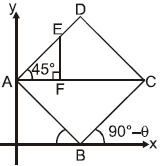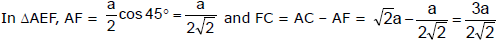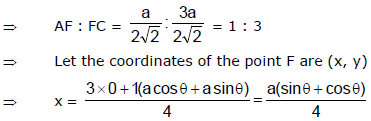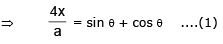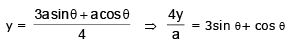...(2)

Form (1) and (2),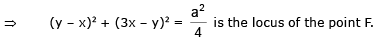Offer running on EduRev: Apply code STAYHOME200 to get INR 200 off on our premium plan EduRev Infinity!

## Mathematics (Maths) Class 11

158 videos|179 docs|161 tests

,

,

,

,

,

,

,

,

,

,

,

,

,

,

,

,

,

,

,

,

,

;